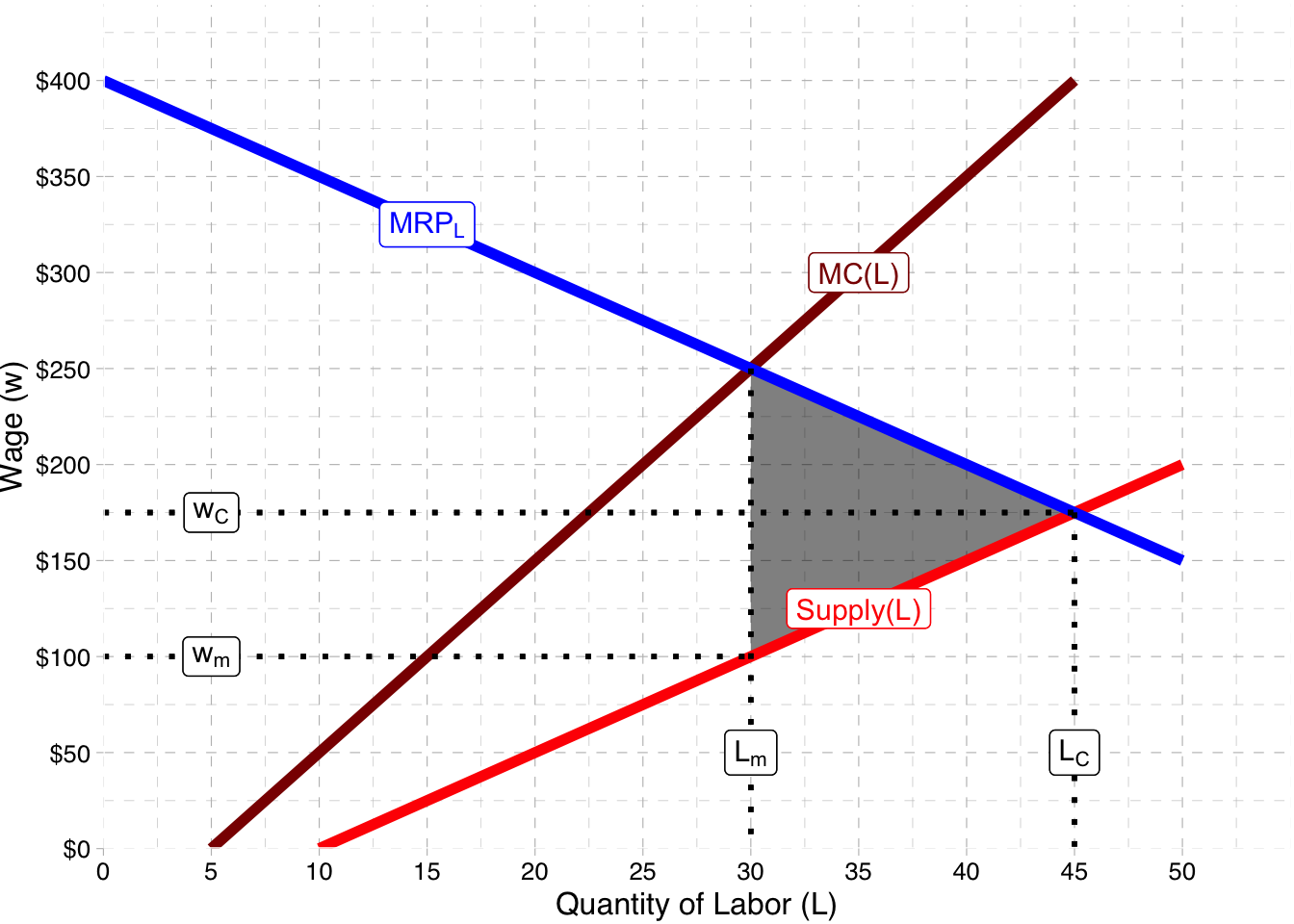Carl’s Coal Mining operates in a remote area. Because of its location, it has monopsony power in the local labor market for miners. Its marginal revenue product of labor is $MRP_L = 400-5L$ where $$L$$ is the total number of miners. The labor supply curve of local miners is $w = 5L-50$ where $$w$$ is the wage (in \$1000’s per miner).

### a. Write a function for the marginal cost of labor.

If we know the (inverse) labor supply function (which we are given), we can simply double the slope to find the marginal cost of labor:

$MC(L)=10L-50$

### b. What quantity of workers will the mine hire, and what wage will it pay its workers?

The optimal quantity of labor to hire for a firm is where its marginal revenue product is equal to the marginal cost of labor:

\begin{aligned} MRP_L&=MC(L)\\ 400-5L&=10L-50\\ 400&=15L-50\\ 450&=15L\\ 30&=L^*\\ \end{aligned}

The firm has monopsony power, so it faces the entire market supply of labor. For $$L^*$$ number of workers, it can pay the lowest wages workers are willing to accept for that quantity, i.e. the labor supply function.

\begin{aligned} w&=5L-50\\ w&=5(30)-50\\ w^*&=100\\ \end{aligned}

### c. What would the quantity of workers be, and what would the wage be, if there was competition among other local mines for labor?

If this was a competitive labor market, with no monopsony power, the firm would be a price-taker of labor, i.e. the supply of labor it faces would be perfectly elastic at the market-determined wage. It would set its marginal revenue product equal to the market wage and hire the quantity of workers for which those values are equal.

\begin{aligned} MRP_L &= w\\ 400-5L &= 5L-50\\ 400&=10L-50\\ 450&=10L\\ 45&=L_c\\ \end{aligned}

Plug this quantity into the (inverse) labor supply function to the find the market wage:

\begin{aligned} w&=5L-50\\ w&=5(45)-50\\ w&=225-50\\ w_C&=175\\ \end{aligned}

### d. Sketch a graph of this market, and be sure to label all of your findings (and show the Deadweight Loss) from Parts A-C.